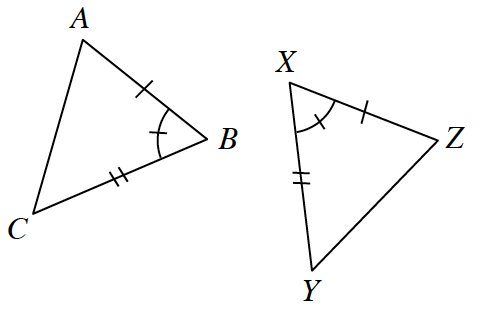### Home > CCG > Chapter 12 > Lesson 12.1.1 > Problem12-12

12-12.

Multiple Choice: Based on the markings in the diagrams at right, which statement is true?

1. $\Delta ABC \cong \Delta XYZ$

1. $\Delta ABC \cong \Delta YXZ$

1. $\Delta ABC \cong \Delta ZXY$

1. $\Delta ABC \cong \Delta ZYX$

1. none of thesec. $ΔABC≅ΔZXY$